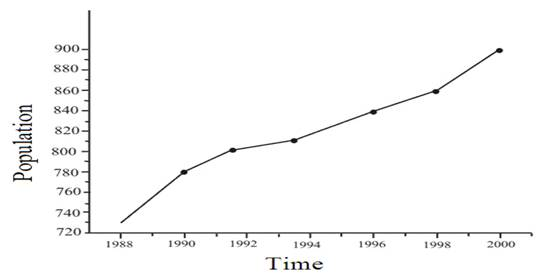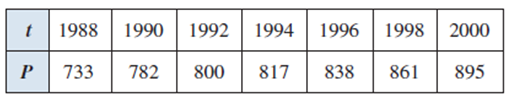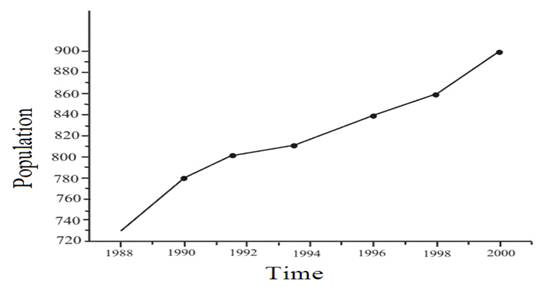# To draw the graph for the given data table.### Precalculus: Mathematics for Calcu...

6th Edition
Stewart + 5 others
Publisher: Cengage Learning
ISBN: 9780840068071### Precalculus: Mathematics for Calcu...

6th Edition
Stewart + 5 others
Publisher: Cengage Learning
ISBN: 9780840068071

#### Solutions

Chapter 2.1, Problem 83E
To determine

## To draw the graph for the given data table.

Expert Solution

The graph for the given data table is:### Explanation of Solution

Given information:The follow graph shows the population of San Jose, California from 1988 to 2000

Start by plotting each individually put years on the x axis and population on the y axis. Notice that the graph on the left has years since 1900.

So, 1988 is 88 (19881900=88) and 2000 is 100 (20001900=100)

To finish the problem, simply connect the dots from left to right to have the graph of P. The problem is not asked for a best-fit line, but rather just to connect the points from the data.

Using advanced grapher then draw the Graph of the function as shown below:### Have a homework question?

Subscribe to bartleby learn! Ask subject matter experts 30 homework questions each month. Plus, you’ll have access to millions of step-by-step textbook answers!